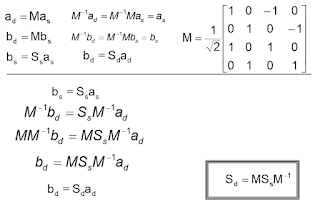## You need to test, we're here to help.

You need to test, we're here to help.

## 17 May 2021

### Converting Single to Mixed-Mode S-ParametersFigure 1: Model of two transmission lines with crosstalk showing the transmission and crosstalk related S-parameters.

We have introduced mixed mode S-parameters and developed a formal structure for handling them. It is now time to discuss converting single-ended S-parameters into mixed-mode S-parameters. This is important because every instrument manufacturer obtains mixed mode S-parameters by first measuring single-ended S-parameters, then converting them mathematically to mixed-mode. This assumes that the interconnects being measured are passive, linear and time invariant.  Let’s begin with our model of two transmission lines with crosstalk shown in Figure 1.

Driving the input port differentially means that the differential voltage at the input is:

a1-a3

Similarly, the differential output is:

b2-b4

Remember that these quantities are vectors with magnitude and phase components, and the math operations are vector operations. With the input and output as defined, we are going to calculate the mixed mode S-parameter SDD21—that is, differential in on port 1 and differential out on port 2.

With reference to Figure 1:

SDD21 = 0.5*(S21-S23+S43-S41)

The b2 term is S21-S23. The b4 term is – (-S43 + S41) = S43 – S41. The factor 0.5 is a normalizing factor.

Applying the same technique using the input S-parameters S11, S13, S33 and S31 (not shown in the figure), we can calculate the differential input SDD11:

SDD11 = 0.5*(S11-S13+S33-S31)

The calculation of all the mixed-mode S-parameters from the single-ended S-parameters using vector algebra can be labor intensive. However, doing the calculation with matrices simplifies the process.Figure 2: The matrix statement that converts single-ended into mixed-mode S-parameters. The “a”s are the input signals, and the “b”s are the output signals.
Let’s look at the basic calculation of differential signals from single-ended signals in matrix form, as seen in Figure 2. If you’re familiar with matrix math, you can read the differential signal input as a1-a3.  Likewise, the differential output is b2-b4.  The matrix form is a more concise way of expressing the relationships, and it contains all the possible mixed signal components.

Notice that again, the port assignments are very important. This is the matrix based on the assignments shown in Figure 1; a different port assignment will require a different matrix.

Single-ended S-parameters relate single-ended inputs into single-ended outputs.  That statement in matrix notation looks like this:

bs = Ss as

Where:  as is the single-ended input vector

bs is the single-ended output vector
Ss is the single-ended S-parameter matrix

Likewise, the relationship for the mixed signal inputs and outputs is:

Where:  ad is the mixed-mode input vector

bd is the mixed-mode output vector
Sd is the mixed-mode S-parameter matrixFigure 3: Solving for mixed-mode S-parameter matrix, Sd, in terms of single-ended S-parameter matrix, Ss.
The goal is to determine the mixed-mode S-parameter matrix from the singled-ended S-parameter matrix.

With some basic matrix math, we can accomplish that as shown in Figure 3.

The M matrix is the transform matrix that relates how single-ended vector elements combine to create the mixed-mode elements. M-1 is the inverse of the transform matrix.Figure 4: Table of mixed-mode S-parameters derived from single-ended S-parameters.
Figure 4 shows the detailed solution to deriving mixed-mode S-parameters from the single-ended S-parameters:

Luckily, if you have a WavePulser 40iX, you do not have to do the math yourself. The instrument does it for you at the touch of a button.

Dr. Eric Bogatin discusses this at more length in his webinar on Mixed Mode S-parameters and TDR Responses.